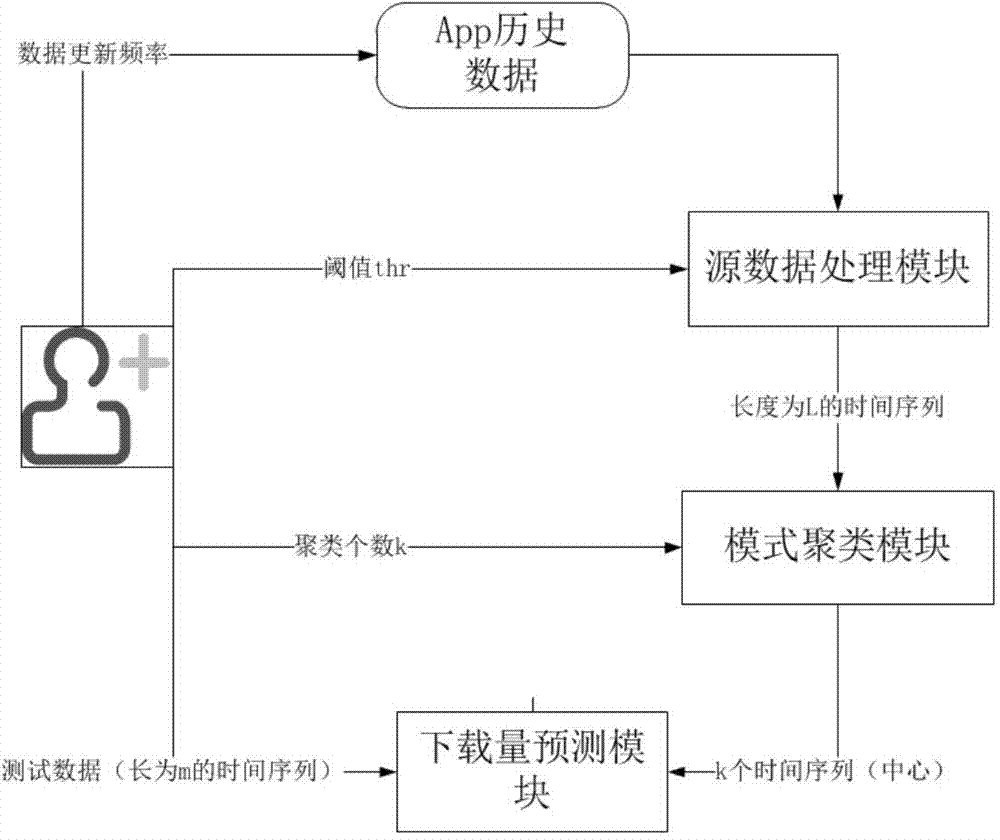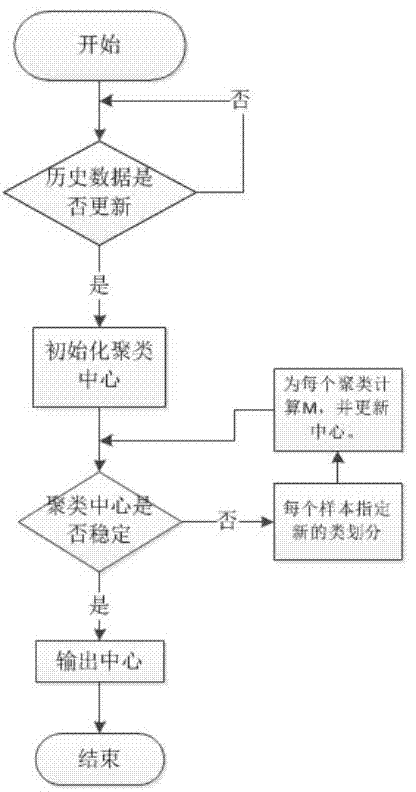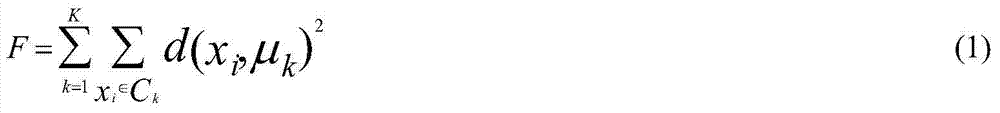## A forecasting method, technology for mobile applications, used in data processing applications, business, instrumentation, etc.

Active Publication Date: 2015-08-19
ZHEJIANG UNIV
7 Cites 0 Cited by

## AI-Extracted Technical Summary

### Problems solved by technology

But currently there is no way to d...
View more

## Abstract

The invention provides a mobile application download prediction method based on cluster. The method comprises: 1) collecting all known app historical data from background data; 2) performing source data processing; 3) and performing mode clustering to predict downloads. The method performs app download prediction in an innovative manner, and is in obvious need in the app field, and has good expansion. The method has very wide application prospect in other E-commerce fields. Based on giving consideration on app download curve features, a K-means algorithm is modified, and solution efficiency is optimized. The whole cluster process can be completed offline, and predicted value calculation of downloads is completed online, so that user experience is optimized.

Application Domain

Commerce

Technology Topic

## Image

•••## Examples

• Experimental program(1)

### Example Embodiment

 Refer to the attached drawings.
 The download forecast method of the present invention includes the following steps:
 1) Obtain historical data of the app to be predicted from the background data, including the downloads of the app in the known m days;
 2) Source data processing. Process the data in step 1) to generate a discrete time series x of length L to represent the download curve of each app. So far, the download curves of all apps form a discrete time series training data set. It includes the following steps:
 The step 2) specifically includes the following steps:
 (1) Given download threshold thr; thr is a manually designated parameter, and the default thr=0.1. Based on the determination of thr, the L of the entire training data set can be calculated 1 And L 2 , Generally adjust thr so ​​that L 1 +L 2 Not less than 2/3 of the original sequence length.
 (2) For each discrete time series x in the discrete time series data set, calculate the corresponding L 1 (x) and L 2 (x), where L 1 (x) means from L p For the first few days, the download volume drops to thr*v for the first time. p The number of days used, correspondingly, L 2 (x) is expressed from L p Starting a few days to the right, the download volume drops to thr*v for the first time p Number of days used; L p Is the day specified in the sequence, v p Is the peak download volume.
 (3) Calculate L in all training data sets 1 (x) and L 2 (x) average value L 2 And L 2. Plus: intercept L before the peak of each discrete sequence 1 Tianhehou L 2 Days of download data, the number of days on the left is less than L 1 When, fill with the data on the right. Correspondingly, the data on the left is used to fill in the lack of data on the right. To ensure that the length of all sequences is L (L=L 1 +L 2 ). So far, the source data has been processed into discrete time series of length L.
 3) Pattern clustering, clustering the discrete time series data set generated in step 2) to obtain k download patterns, which specifically includes the following steps:
 (1) Set the number k of pattern clusters in the training data set; k is a manually designated parameter, and the default k=6, and its specific value is adjusted according to the clustering effect.
 (2) Randomly designate k curves as the centers of k clusters from the training data set, and calculate the curve distance d(x,c) from each non-central discrete time series x to k centers;
 d(x,c) represents the distance between x and a certain cluster center, and c specifically refers to the cluster center (center). According to d(x,c), the discrete sequence is divided to the nearest cluster center. class.
 (3) Update cluster center k, the target of each cluster center update becomes to minimize F;
 Under a given class division, the goal of cluster update is to minimize the sum of squared distances from each discrete time series in the class to the class center.
 F = X k = 1 K X x i A C k d ( x i , μ k ) 2 - - - ( 1 )
 Where μ k , C k These are the center of the kth class and the curve belonging to the kth class.
 According to formula (1), the value of each k-th category update can be derived:
 μ k * = arg min μ X x i A C k d ( x i , μ ) 2 - - - ( 2 )
 among them Is the value of the center of the k-th category after the update.
 ( 4 ) α = x i T μ | | xi | | 2
 α is the scaling factor of the ordinate of another discrete sequence.
 μ k * = arg min μ 1 | | μ | | 2 X x i A C k | | ( x i x i T | | xi | | 2 - I ) μ | | 2 - - - ( 3 )
 Further formula 4 can be derived from formula 3:
 μ k * = arg min μ 1 | | μ | | 2 μ T X x i A C k ( I - x i x i T | | xi | | 2 ) μ - - - ( 4 )
 make M = X x i A C k ( I - x i x i T | | x i | | 2 ) , Can get The final calculation method:
 μ k * = arg min μ μ T Mμ | | μ | | 2
 therefore, It is the eigenvector corresponding to the smallest eigenvalue of matrix M.
 Among them, T means transpose the matrix, C k Represents the set of curves belonging to the kth category in the current division. x i Represents the i-th item in the discrete sequence x, the value of M is only in x i Correlation, no specific physical meaning, I is a constant, corresponding to the i-th item in x, and u represents the matrix formed by various centers.
 The algorithm used for pattern clustering is similar to k-means, and each iteration is divided into two steps. Unlike the Euclidean distance used by k-means, the curve distance calculation method in the definition is used here.
 The update of the clustering center by the way of finding the feature vector makes the realization of the algorithm easier, and also effectively reduces the complexity of the solution.
 (1) Given an app, the download curve of the first m days is a discrete time series test of length m, and the calculation test and each center (the cluster center itself is a discrete sequence of length L) constituted by the first m days Cosine similarity of discrete sequence, select the most similar class center c;
 (2) The following L-m-day total download volume pred is predicted as follows:
 pred = X j = 1 m test j X j = 1 m c j * X j = 1 + m L c j ,
 Among them, c represents the selected most similar class center, then cj represents the jth item of the discrete sequence c.

## PUM## Description & Claims & Application Information

We can also present the details of the Description, Claims and Application information to help users get a comprehensive understanding of the technical details of the patent, such as background art, summary of invention, brief description of drawings, description of embodiments, and other original content. On the other hand, users can also determine the specific scope of protection of the technology through the list of claims; as well as understand the changes in the life cycle of the technology with the presentation of the patent timeline. Login to view more.
Who we serve
• R&D Engineer
• R&D Manager
• IP Professional
Why Eureka